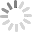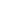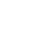## Free, outstanding support from WebAssist and your colleagues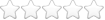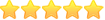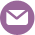# eCart calculation

Thread began 10/15/2009 3:34 pm by Fritz Stickers | Last modified 11/24/2009 1:36 pm by Eric Mittman | 2633 views | 7 replies |

##10/15/2009 3:34 pm  |  #1 Fritz Stickers

### eCart calculation

Hi,

The price for a product increase with 2,00 euro when the size increase with 5 cm.
The minimum price is 6,00 euro for 15 cm, so 20 cm=8,00; 25 cm=10,00; 30 cm=12,00.
WA_eCart calculation : [Price]+(((([Size]-15)/5)*2))

Now I want the price increase with just 1,00 euro from size 35 cm, so that 35 cm=13 euro.

How do I have to make the calculation ?

Regards,
Geert.

##10/16/2009 2:19 pm  |  #2 Eric Mittman

I was looking into this one for a moment and I think I have a calculation that will work for you.

The idea I was working with is that there is a max of 6 euro that will be added to the minimum price if the size is 30 or greater using your posted calculation. So I just made a calculation that checks if it is over 30cm and if so add 1 euro for each 5 cm over 30cm, then also add 6 euro to account for the maximum charge for the first calculation. If it is 30cm or less then just use the original calculation. Here is the calculation code:

[Price]+ (abs([Size] > 30) * ((([Size]-30)/5) + 6)) + (abs([Size] <= 30) * ((([Size]-15)/5)*2))

Please give this a try and let us know if it works for you. Sorry if my explanation of the code is confusing.

##10/17/2009 6:28 am  |  #3 Fritz Stickers

Thanks Eric,

Calculation works fine !

Geert.

##10/19/2009 3:28 pm  |  #4 Eric Mittman

That is good to hear, I was hoping it would work for you. It took me a minute or two to review it and test it.

##11/24/2009 8:50 am  |  #5 Fritz Stickers

Hi,

I want to change the calculation a little bit :

[Price]+ (abs([Size] > 30) * ((([Size]-30)/5) + 6)) + (abs([Size] <= 30) * ((([Size]-15)/5)*2))

I want also the price decrease 1 euro if size is 10 cm.

10 cm = 5,00 (-1,00)
15 cm = 6,00 (= 6,00)
20 cm = 8,00 (+2,00)
25 cm = 10,00 (+4,00)
30 cm = 12,00 (+6,00)
35 cm = 13,00 (+7,00)
40 cm = 14,00 (+8,00)
45 cm = 15,00 (+9,00)

I tried a lot of combinations for the calculation, but non of them works.
([Price]+ (abs([Size] = 10) * (([Size]-15)/5)) + (abs([Size] <= 30) * ((([Size]-15)/5)*2)) + (abs([Size] > 30) * ((([Size]-30)/5) + 6))))

Can you give it a try ?

Geert.

##11/24/2009 10:58 am  |  #6 Fritz Stickers

I found it at last :

- 1 euro < 15 cm
+ 2,00 euro = 20,25 of 30 cm
+ 1,00 euro > 30 cm

([Price]+ (abs([Size] < 15) * (([Size]-15)/5))
+ (abs([Size] = 20) * ((([Size]-15)/5)*2))
+ (abs([Size] = 25) * ((([Size]-15)/5)*2))
+ (abs([Size] = 30) * ((([Size]-15)/5)*2))
+ (abs([Size] > 30) * ((([Size]-30)/5) + 6))))

##11/24/2009 11:46 am  |  #7 Office Guy-172461

Thanks to both of you for sharing your formulas.

Calculation for price increase based on size
169/

##11/24/2009 1:36 pm  |  #8 Eric Mittman

Nice write up on this, I bet this will come in handy for others out there. Thanks.

## Build websites with a little help from your friends

Your friends over here at WebAssist! These Dreamweaver extensions will assist you in building unlimited, custom websites.

## Build websites from already-built web applications

These out-of-the-box solutions provide you proven, tested applications that can be up and running now.  Build a store, a gallery, or a web-based email solution.

## Everything else!

Close Window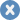Account or customer service questions?

Need technical support?

ContentLayoutEase of use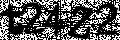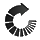We do not respond to comments submitted from this page directly, but we do read and analyze any feedback and will use it to help make your experience better in the future.

Close Window## We were unable to retrieve the attached file

Close Window### Attach and remove files

• Text Formatting
• Insert Element
• Block Formatting
• Cancel
Close Window#### Enter the URL you would like to link to in your post

Close Window#### This is how you use right click RTF editing

Enable right click RTF editing option allows you to add html markup into your tutorial such as images, bulleted lists, files and more...-- click to close --